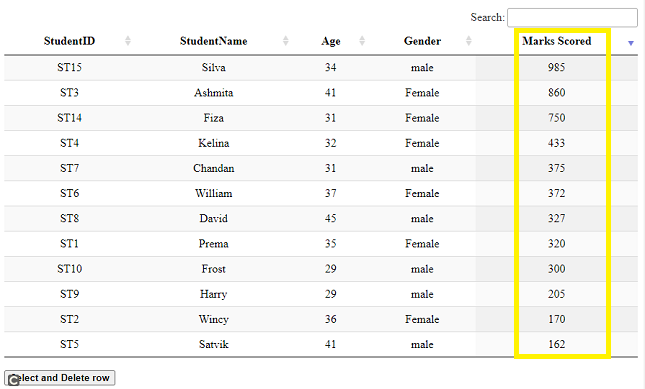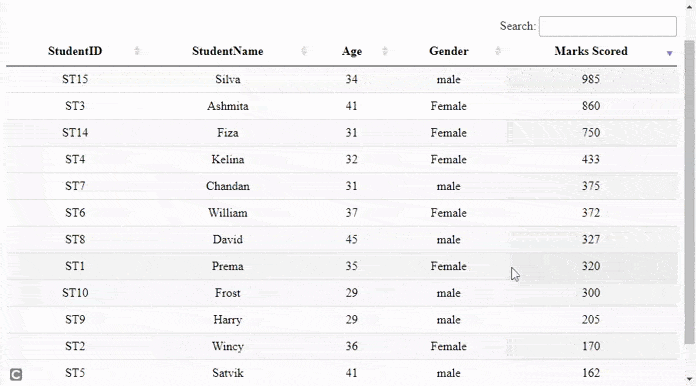# How to implement single row select and delete using DataTables plugin ?

• Last Updated : 31 May, 2021

DataTables are a modern jQuery plugin for adding interactive and advanced controls to HTML tables for a webpage. It is a very simple-to-use plug-in with a variety of options for the developer’s custom changes as per the application’s need. The plugin’s features include pagination, sorting, searching, and multiple-column ordering.

In this article, we will demonstrate the implementation of single row deletion after selecting the row using the DataTables plugin.

Approach: In the following example, DataTables uses the student details from the HTML table as the main source. Each row in the table shows details for one student’s information.

• A DataTable is initialized.
• The developer can set the features of paging or searching as per the need as shown in the script part of the code.
• The table is set in the descending order of student’s marks just to show the ordering usage.
• Check if the row selected by the user has class “selected” and then remove it.
• If the class is not “selected” then it is removed from all other rows in the table and the class is added to the row being selected.
• On button click, remove the row along with setting the draw() API to false.
• The draw() API is used to reflect the changes after an action in the table.

The pre-compiled files which are needed to implement are

CSS:

`https://cdn.datatables.net/1.10.22/css/jquery.dataTables.min.css`

JavaScript:

```https://code.jquery.com/jquery-3.5.1.js
https://cdn.datatables.net/1.10.22/js/jquery.dataTables.min.js```

Example: The following example demonstrates the above approach showing single row select and then delete the row.

## HTML

 ` ` `<``html``> ` ` `  `<``head``> ` ` `  `    ``<``meta` `content="``initial-scale``=``1``, ``maximum-scale``=``1``, ` `                   ``user-scalable``=``0``" ``name``=``"viewport"` `/> ` `    ``<``meta` `name``=``"viewport"` `content``=``"width=device-width"` `/> ` ` `  `    ``` `    ``<``link` `rel``=``"stylesheet"` `href``= ` `"https://cdn.datatables.net/1.10.22/css/jquery.dataTables.min.css"` `/> ` ` `  `    ``` `    ``<``script` `type``=``"text/javascript"`  `        ``src``=``"https://code.jquery.com/jquery-3.5.1.js"``> ` `    `` ` ` `  `    ``` `    ``<``script` `type``=``"text/javascript"` `src``= ` `"https://cdn.datatables.net/1.10.22/js/jquery.dataTables.min.js"``> ` `    `` ` ` `  `    ``<``style``> ` `        ``td { ` `            ``text-align: center; ` `        ``} ` `    `` ` ` ` ` `  `<``body``> ` `    ``<``h2``>Delete single row using DataTables plugin ` ` `  `    ``` `    ``<``table` `id``=``"tableID"` `class``=``"display"` `style``=``"width:100%"``> ` `        ``<``thead``> ` `            ``<``tr``> ` `                ``<``th``>StudentID ` `                ``<``th``>StudentName ` `                ``<``th``>Age ` `                ``<``th``>Gender ` `                ``<``th``>Marks Scored ` `            `` ` `        `` ` ` `  `        ``<``tbody``> ` `            ``<``tr``> ` `                ``<``td``>ST1 ` `                ``<``td``>Prema ` `                ``<``td``>35 ` `                ``<``td``>Female ` `                ``<``td``>320 ` `            `` ` `            ``<``tr``> ` `                ``<``td``>ST2 ` `                ``<``td``>Wincy ` `                ``<``td``>36 ` `                ``<``td``>Female ` `                ``<``td``>170 ` `            `` ` `            ``<``tr``> ` `                ``<``td``>ST3 ` `                ``<``td``>Ashmita ` ` `  `                ``<``td``>41 ` `                ``<``td``>Female ` `                ``<``td``>860 ` `            `` ` `            ``<``tr``> ` `                ``<``td``>ST4 ` `                ``<``td``>Kelina ` `                ``<``td``>32 ` `                ``<``td``>Female ` `                ``<``td``>433 ` `            `` ` `            ``<``tr``> ` `                ``<``td``>ST5 ` `                ``<``td``>Satvik ` `                ``<``td``>41 ` `                ``<``td``>male ` `                ``<``td``>162 ` `            `` ` `            ``<``tr``> ` `                ``<``td``>ST6 ` `                ``<``td``>William ` `                ``<``td``>37 ` `                ``<``td``>Female ` `                ``<``td``>372 ` `            `` ` `            ``<``tr``> ` `                ``<``td``>ST7 ` `                ``<``td``>Chandan ` `                ``<``td``>31 ` `                ``<``td``>male ` `                ``<``td``>375 ` `            `` ` `            ``<``tr``> ` `                ``<``td``>ST8 ` `                ``<``td``>David ` `                ``<``td``>45 ` `                ``<``td``>male ` `                ``<``td``>327 ` `            `` ` `            ``<``tr``> ` `                ``<``td``>ST9 ` `                ``<``td``>Harry ` `                ``<``td``>29 ` `                ``<``td``>male ` `                ``<``td``>205 ` `            `` ` `            ``<``tr``> ` `                ``<``td``>ST10 ` `                ``<``td``>Frost ` `                ``<``td``>29 ` `                ``<``td``>male ` `                ``<``td``>300 ` `            `` ` ` `  `            ``<``tr``> ` `                ``<``td``>ST14 ` `                ``<``td``>Fiza ` `                ``<``td``>31 ` `                ``<``td``>Female ` `                ``<``td``>750 ` `            `` ` `            ``<``tr``> ` `                ``<``td``>ST15 ` `                ``<``td``>Silva ` `                ``<``td``>34 ` `                ``<``td``>male ` `                ``<``td``>985 ` `            `` ` ` `  `        `` ` `    `` ` `    ``<``br` `/> ` ` `  `    ``<``button` `id``=``"btnID"``><``b``>Select and Delete row ` ` `  `    ``<``script``> ` ` `  `            ``/* Initialization of datatables */ ` `            ``\$(document).ready(function () { ` ` `  `                ``// Paging and other information are ` `                ``// disabled if required, set to true ` `                ``var mytableVar = \$('#tableID').DataTable({ ` `                    ``"paging": false, ` `                    ``"info": false ` `                ``}); ` ` `  `                ``// Ordering the table in descending ` `                ``// order of students marks ` `                ``mytableVar.order([[4, 'desc']]).draw(false); ` ` `  ` `  `                ``\$('#tableID tbody').on('click', 'tr', function () { ` ` `  `                    ``// Check for 'selected' class and remove ` `                    ``if (\$(this).hasClass('selected')) { ` `                        ``\$(this).removeClass('selected'); ` `                    ``} ` `                    ``else { ` `                        ``mytableVar.\$('tr.selected').removeClass('selected'); ` `                        ``\$(this).addClass('selected'); ` `                    ``} ` `                ``}); ` ` `  `                ``// On button click, remove the row with setting ` `                ``// the draw() to false ` `                ``// draw() API is used to reflect the changes ` `                ``// after a action ` `                ``\$('#btnID').click(function () { ` `                    ``mytableVar.row('.selected').remove().draw(false); ` `                ``}); ` `            ``}); ` `    `` ` ` ` ` `  ` `

Output:

• Before Clicking the button: Notice that the marks scored by the students in the last column are in descending order.• After Clicking the button: The following output shows that the row for student name “William” is deleted.My Personal Notes arrow_drop_up
Recommended Articles
Page :